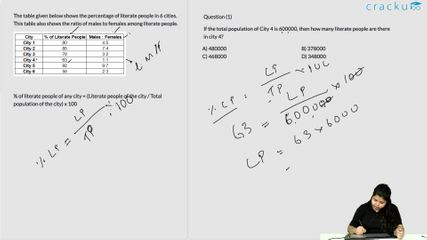### 2017 SSC CGL 09 Aug Shift-2 Question 97

Instructions

The table given below shows the percentage of literate people in 6 cities. This table also shows the ratio of males to females among literate people.

Question 97

# If the total population of City 4 is 600000, then how many literate people are there in city 4?

Solution

Total population of City 4 = 600,000

=> Literate population in city 4 = $$\frac{63}{100}\times600,000$$

= $$378,000$$

=> Ans - (B)

### View Video Solution